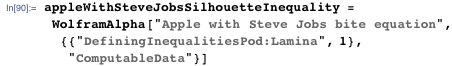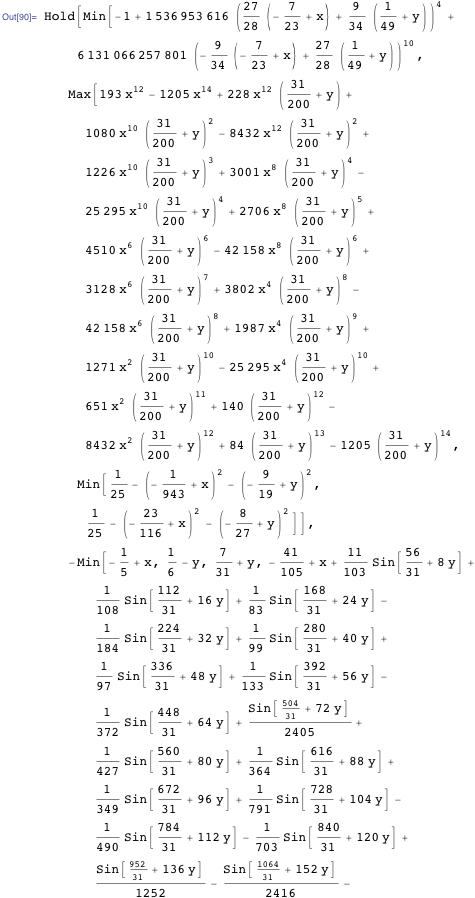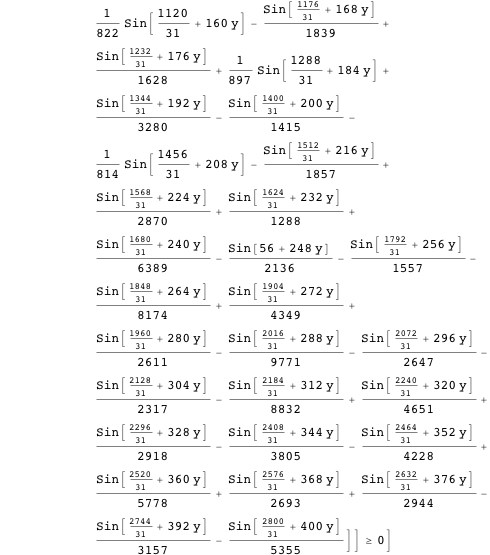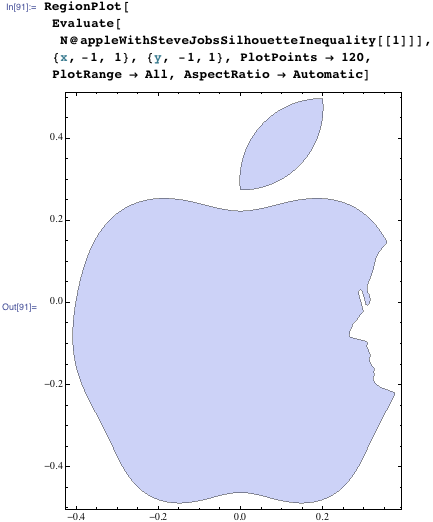Browse by Topic
Related Topics

# Even More Formulas… for Everything—From Filled Algebraic Curves to the Twitter Bird, the American Flag, Chocolate Easter Bunnies, and the Superman Solid

This blog post is the continuation of my last two posts (1, 2) about formulas for curves. So far, we have discussed how to make plane curves that are sketches of animals, faces, fictional characters, and more. In this post, we will discuss the constructions of some filled curves (laminae).

Here are some of the non-mathematical laminae that Wolfram|Alpha knows closed-form equations for: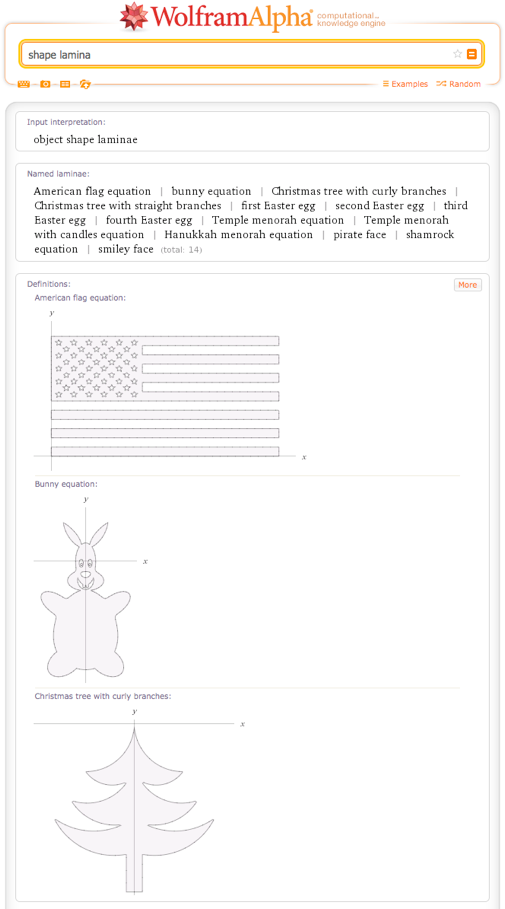Assume we want a filled curve instead of just the curve itself. For closed curves, say the James Bond logo, we could just take the curve parametrizations and fill the curves. As a graphics object, filling a curve is easy to realize by using the FilledCurve function.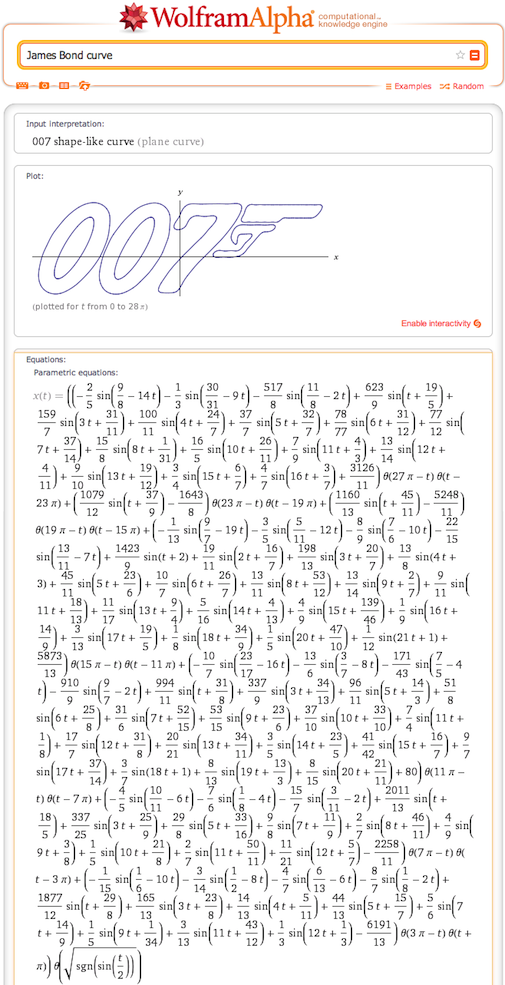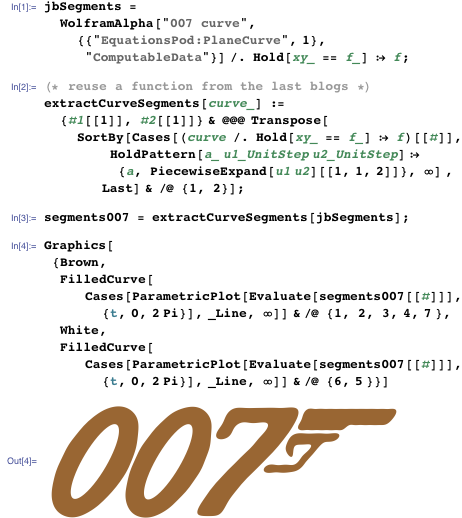For the original curves, we had constructed closed-form Fourier series-based parametrizations. While the FilledCurve function yields a visually filled curve, it does not give us a closed-form mathematical formula for the region enclosed by the curves. We could write down contour integrals along the segment boundaries in the spirit of Cauchy’s theorem to differentiate the inside from the outside, but this also does not result in “nice” closed forms. So, for filled curves, we will use another approach, which brings us to the construction of laminae for various shapes.

The method we will use is based on constructive solid geometry. We will build the laminae from simple shaped regions that we first connect with operations such as AND or OR. In a second step, we will convert the logical operations by mathematical functions to obtain formulas of the form f(x, y) > 0 for the region that we want to describe. The method of conversion from the logical formula to an arithmetic function is based on Rvachev’s R-function theory.

Let’s now construct a geometrically simple shape using the just-described method: a Mitsubishi logo-like lamina, here shown as a reminder of how it looks.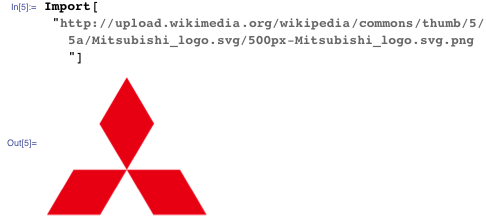As this sign is obviously made from three rhombi, we define a function polygonToInequality that describes the interior of a single convex polygon. A point is an interior point if it lies on the inner side of all the line segments that are the boundaries of the polygon. We test the property of being inside by forming the scalar product of the normals of the line segments with the vector from a line segment’s end point to the given point.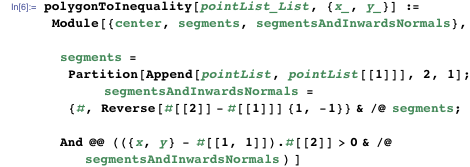It is simple to write down the vertices of the three rhombi, and so a logical formula for the whole logo.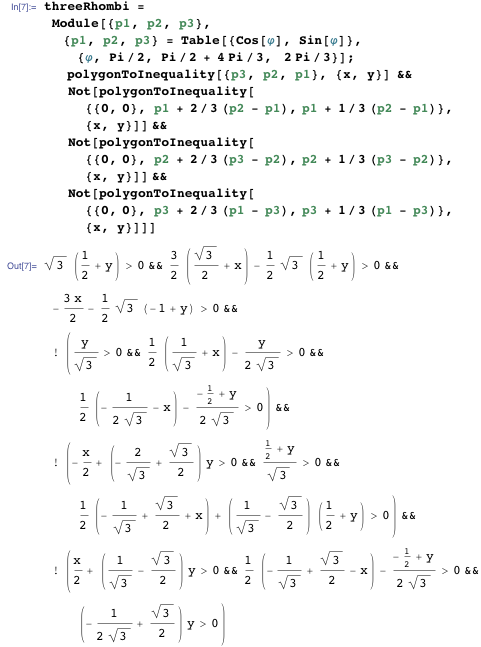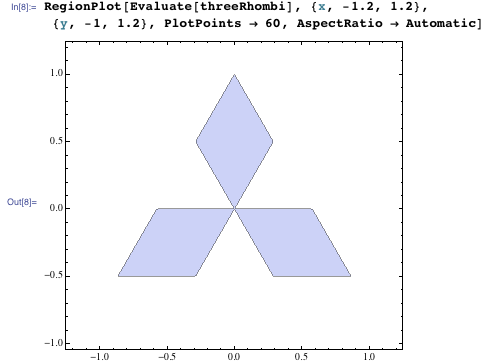The last equation can be considerably simplified.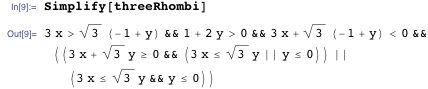The translation of the logical formula into a single inequality is quite simple: we first write all inequalities with a right-hand side of zero and then translate the Or function to the Max function and the And function to the Min function. This is the central point of the Rvachev R-function theory. By using more complicated translations, we could build right-hand sides of a higher degree of smoothness, but for our purposes Min and Max are sufficient. The points where the right-hand side of the resulting inequality is greater than zero we consider part of the lamina, otherwise the points are outside. In addition to just looking nicer and more compact, the single expression, as compared to the logical formula, evaluates to a real number everywhere. This means, in addition to just a yes/no membership of a point {x,y}, we have actual function values f(x, y) available. This is an advantage, as it allows for plotting f(x, y) over an extended region. It also allows for a more efficient plotting than the logical formula because function values around f(x, y) = 0 can be interpolated.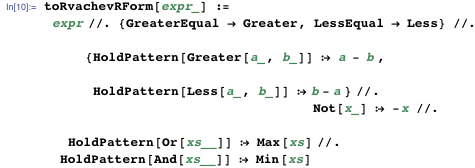So we obtain the following quite simple right-hand side for the inequality that characterizes the Mitsubishi logo.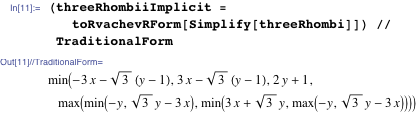And the resulting image looks identical to the one from the logical formula.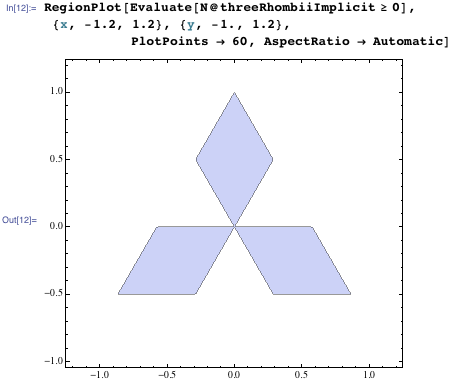Plotting the right-hand side for the inequality as a bivariate function in 3D shows how the parts of the inequality that are positive emerge from the overall function values.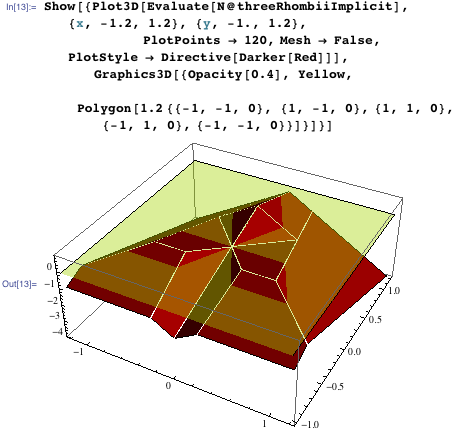Now, this type of construction of a region of the plane through logical formulas of elementary regions can be applied to more regions and to regions of different shapes, not necessarily polygonal ones. In general, if we have n elementary building block regions, we can construct as many compound regions as there are logical functions in n variables. The function BooleanFunction enumerates all these 2^2^n possibilities. The following interactive demonstration allows us to view all 65,536 configurations for the case of four ellipses. We display the logical formula (and some equivalent forms), the 2D regions described by the formulas, the corresponding Rvachev functions, and the 3D plot of the Rvachev R-function. The region selected is colored yellow.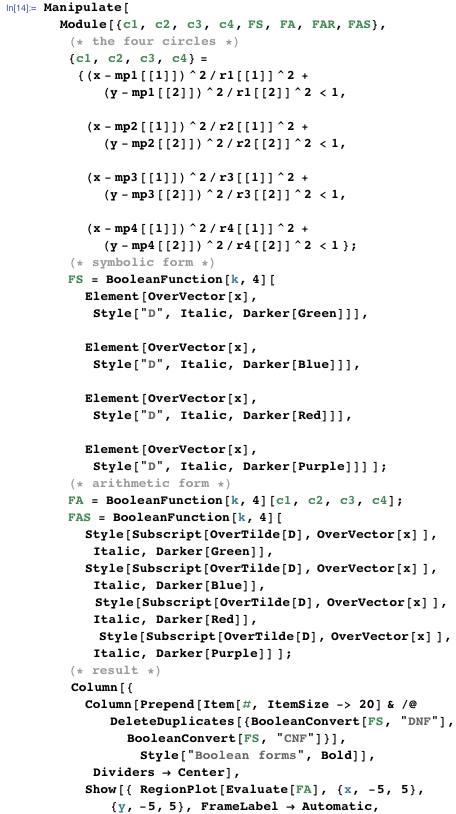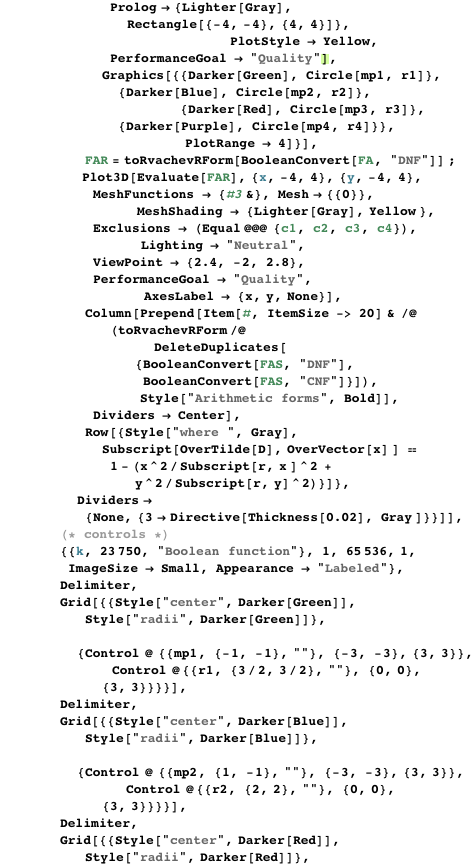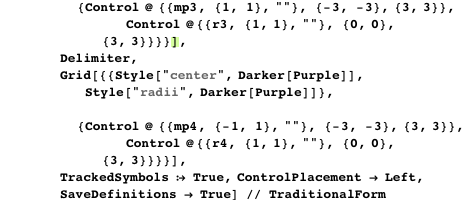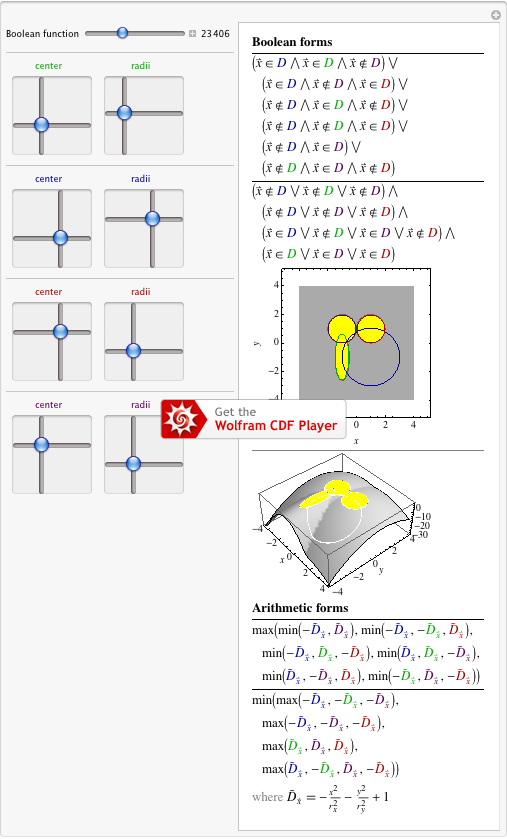Cutting out a region from not just four circles, but seven, we can obtain the Twitter bird. Here is the Wolfram|Alpha formula for the Twitter bird. (Worth a tweet?)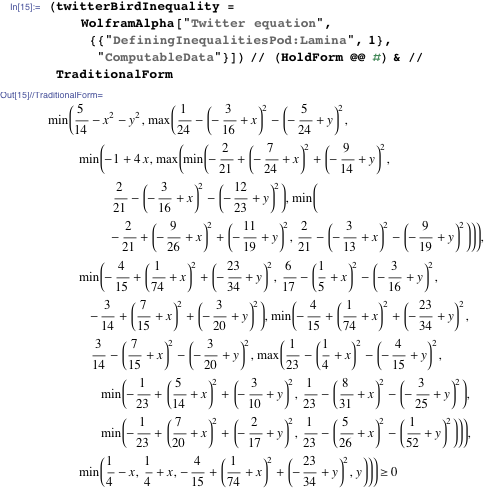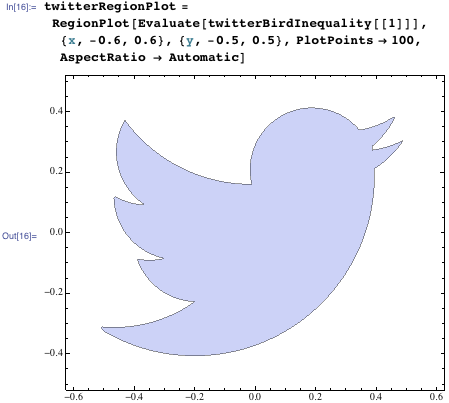By drawing the zero-curves of all of the bivariate quadratic polynomials that appear in the Twitter bird inequality as arguments of max and min, the disks of various radii that were used in the construction become obvious. The total bird consists of points from seven different disks. Some more disks are needed to restrict the parts used from these six disks.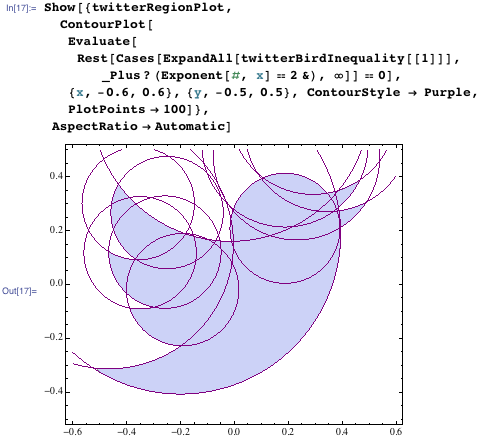Here are two 3D versions of the Twitter bird as 3D plots. As the left-hand side of the Rvachev R-equation evaluates to a number, we use this number as the z value (possibly modified) in the plots.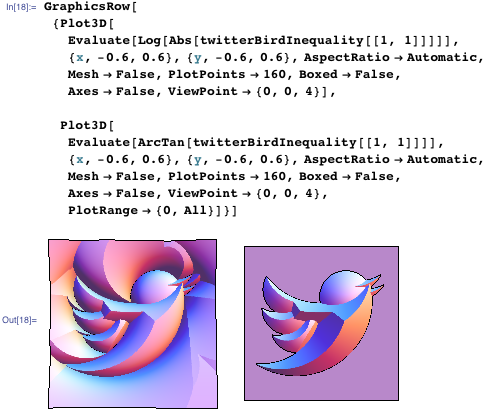We can also use the closed-form equation of the Twitter bird to mint a Twitter coin.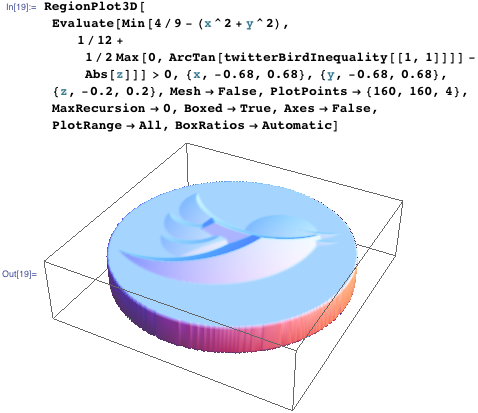The boundary of the laminae described by Rvachev-R functions has the form f(x, y) = 0. Generalizing this to f(x, y) = g(z) naturally extrudes the 2D shape into 3D, and by using a function that increases with |z|, we obtain closed 3D surfaces. Here this is done for g(z) ~ (z^2) for the Twitter bird (we also add some color and add a cage to confine the bird). The use g(z) ~ (z^2) means z ~ ± f(x y)^(1/2) at the boundaries, and the infinite slope of the square root functions gives a smooth bird surface near the z = 0 plane.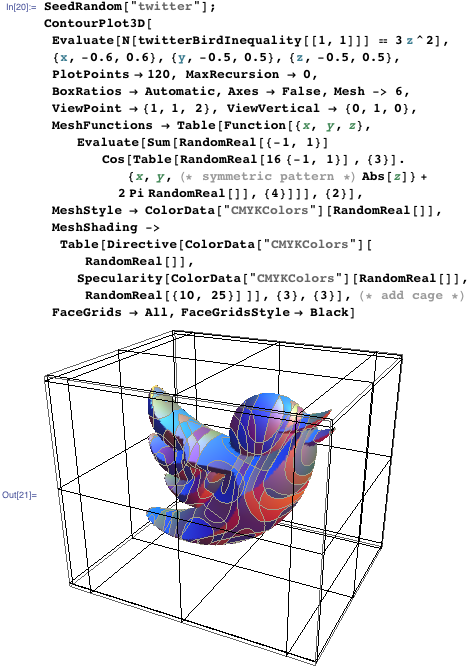Now we will apply the above-outlined construction idea to a slightly more complicated example: we will construct an equation for the United States’ flag. The most complicated-looking part of the construction is a single copy of the star. Using the above function polygonToInequality for the five triangle parts of the pentagram and the central pentagon, we obtain after some simplification the following form for a logical function describing a pentagram.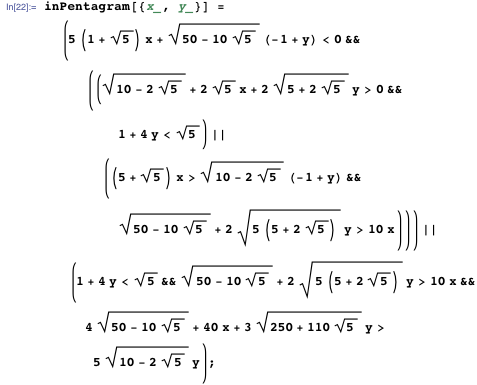Here is the pentagram shown, as well the five lines that occur implicitly in the defining expression of the pentagram.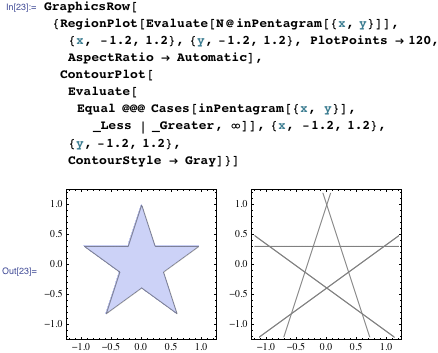The detailed relative sizes of the stars and stripes are specified in Executive Order 10834 (“Proportions and Sizes of Flags and Position of Stars”) of the United States government. Using the data from this document and assuming a flag of height 1, it is straightforward to encode the non-white parts of the US flag in the following manner. For the parallel horizontal stripes we use a sin(α y) (with appropriately chosen α) construction. The grid of stars in the left upper corner of the flag is made from two square grids, one shifted against the other (a 2D version of an fcc lattice). The Mod function allows us to easily model the lattice arrays.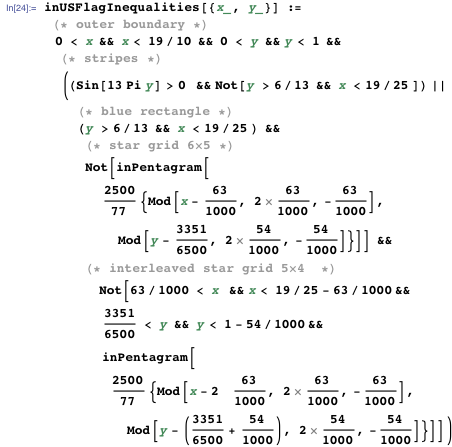This gives the following closed-form formula for the US flag. Taking the visual complexity of the flag into account, this is quite a compact description.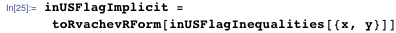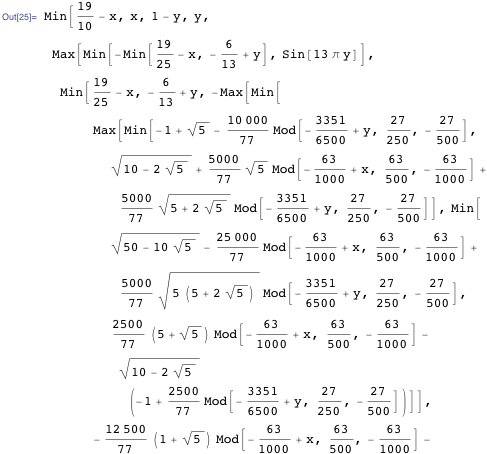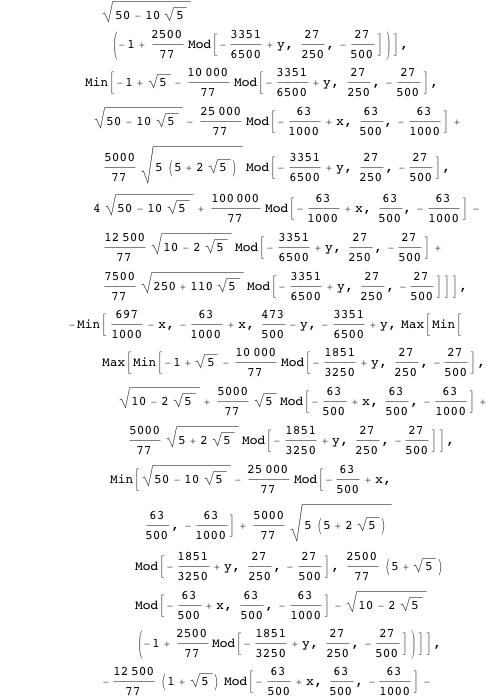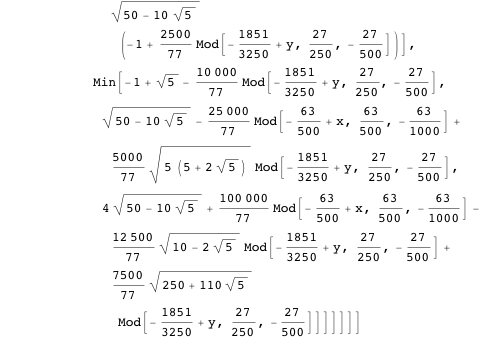Making a plot of this formula gives—by construction—the American flag.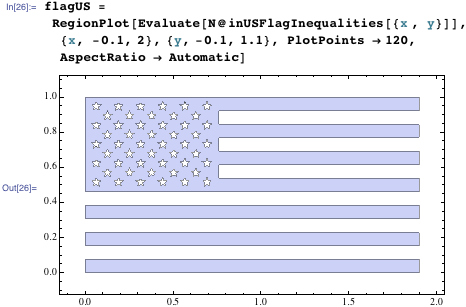We can apply a nonlinear coordinate transformation to the inequality to let the flag flow in the wind.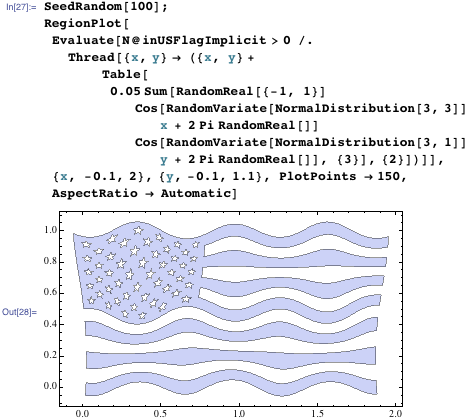And using a more quickly varying map, we can construct a visual equivalent of Jimi Hendrix‘s “Star-Spangled Banner” from the Rainbow Bridge album.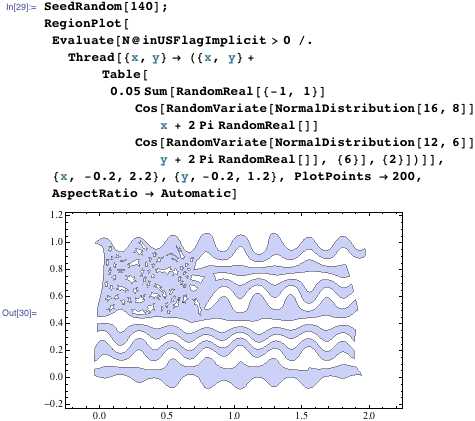As laminae describe regions in 2D, we can identify the plane with the complex plane and carry out conformal maps on the complex plane, such as for the square root function or the square.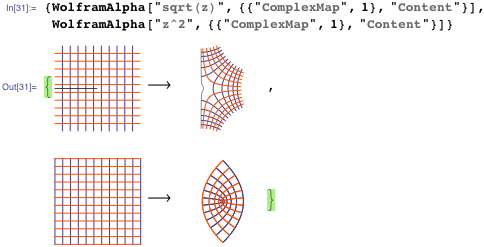Here are the four maps that we will apply to the flag.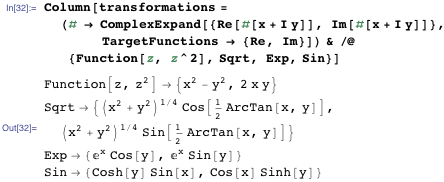And these are the conformally mapped flags.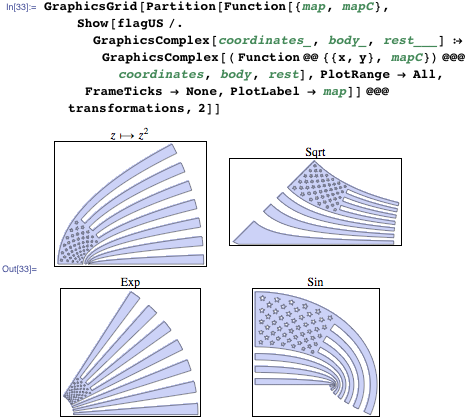The next interactive demonstration applies a general power function z -> (shift + scale z)α to the plane containing the flag. (For some parameter values, the branch cut of the power function can lead to folded over polygons.)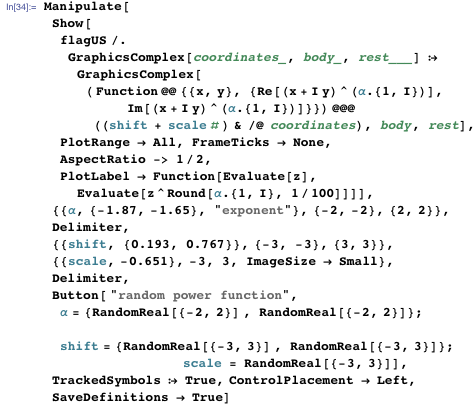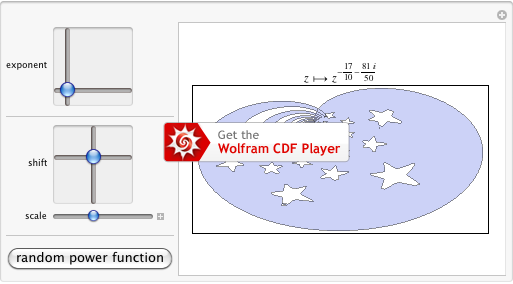So far we have used circles and polygons as the elementary building blocks for our lamina. It is straightforward to use more complicated shapes. Let’s model a region of the plane that approximates the logo of the largest US company—the apple from Apple. As this is a more complicated shape, calculating an equation that describes it will need a bit more effort (and code). Here is an image of the shape to be approximated.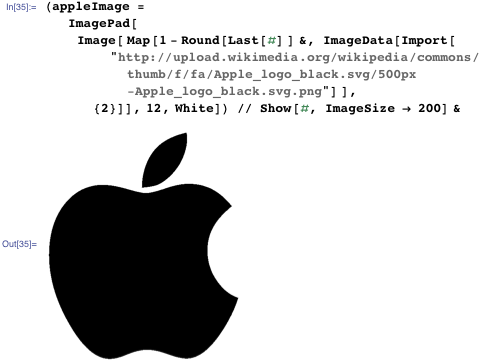So, how could we describe a shape like an apple? For instance, one could use osculating circles and splines (see this blog entry). Here we will go another route. Algebraic curves can take a large variety of shapes. Here are some examples:

Stone–Weierstrass theorem, which guarantees that any continuous function can be approximated by polynomials.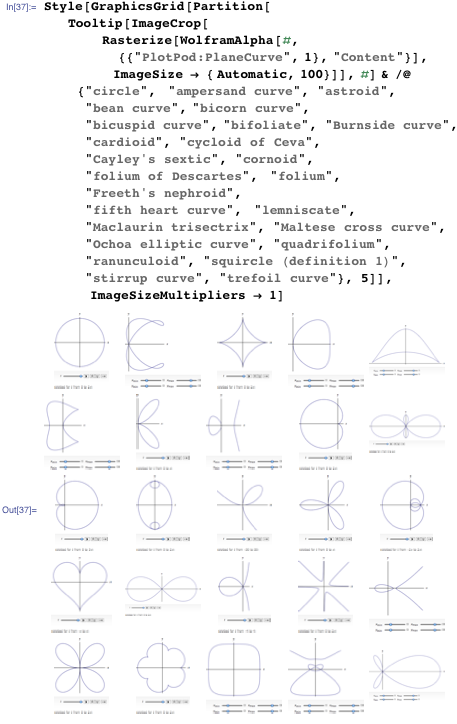We look for an algebraic curve that will approximate the central apple shape. To do so, we first extract again the points that form the boundary of the apple. (To do this, we reuse the function pointListToLines from the first blog post of this series, mentioned previously.)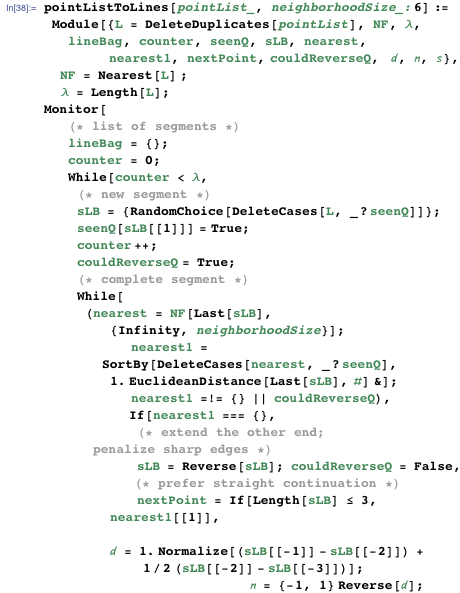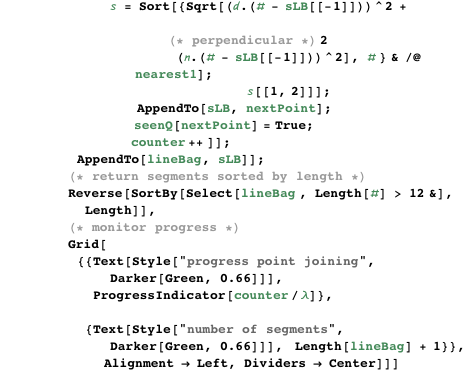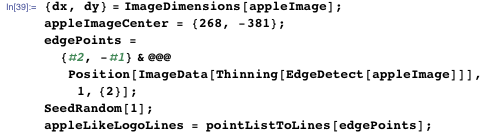We assume that the core apple shape is left-right symmetric and select points from the left side (meaning the side that does not contain the bite). The following Manipulate allows us to quickly to locate all points on the left side of the apple.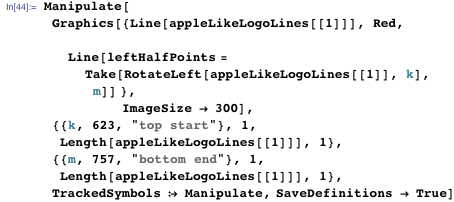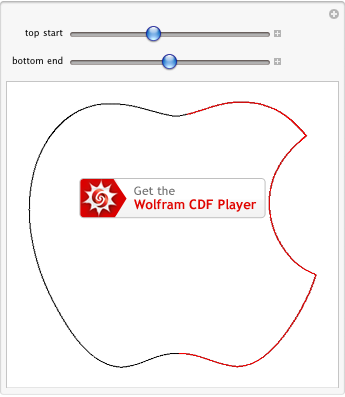To find a polynomial p (x, y) = 0 that describes the core apple, we first use polar coordinates (with the origin at the apple’s center) and find a Fourier series approximation of the apple’s boundary in the form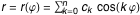. The use of only the cosine terms guarantees the left-right symmetry of the resulting apple.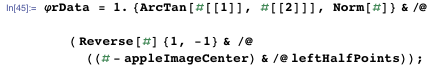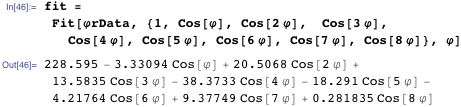We rationalize the resulting approximation and find the corresponding bivariate polynomial in Cartesian coordinates using GroebnerBasis. After expressing the cos(kφ) terms in terms of just cos(φ) and sin(φ), we use the identity cos(φ)^2+sin(φ)^2 = 1 to eliminate cos(φ) and sin(φ) to obtain a single polynomial equation in x and y.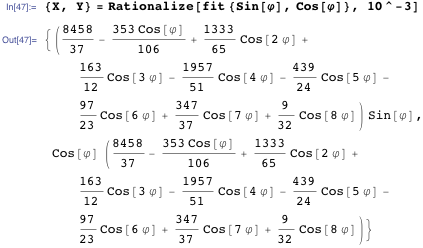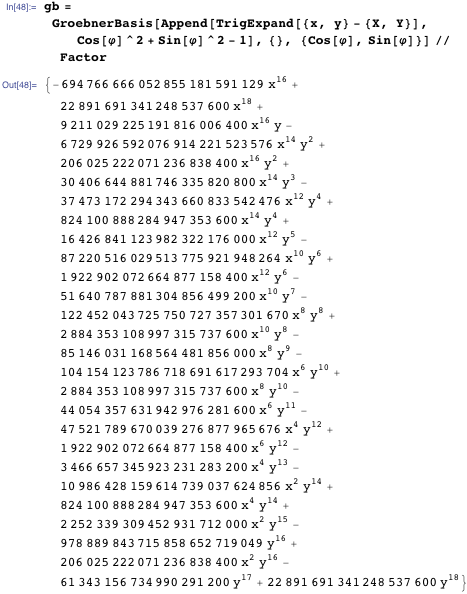As we rounded the coefficients, we can safely ignore the last digits in the integer coefficients of the resulting polynomial and so shorten the result.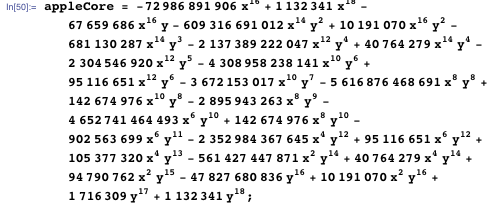Here is the resulting apple as an algebraic curve.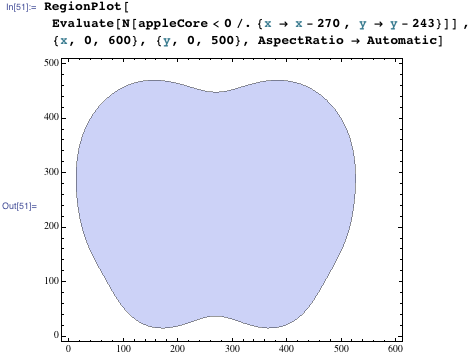Now we need to take a bite on the right-hand side and add the leaf on top. For both of these shapes, we will just use circles as the geometric shapes. The following interactive Manipulate allows the positioning and sizing of the circles, so that they agree with the Apple logo. The initial values are chosen so that the circles match the original image boundaries. (We see that the imported image is not exactly left-right symmetric.)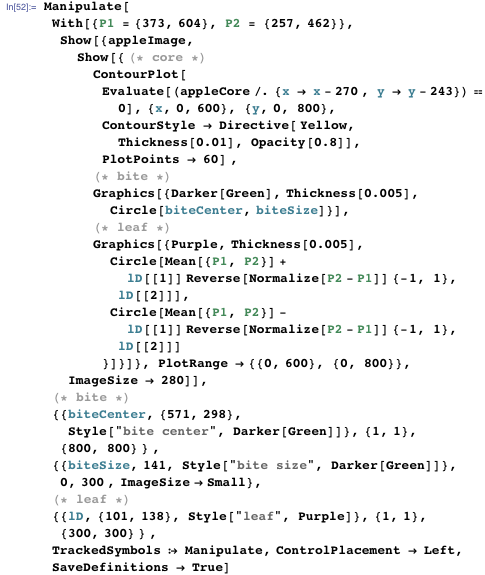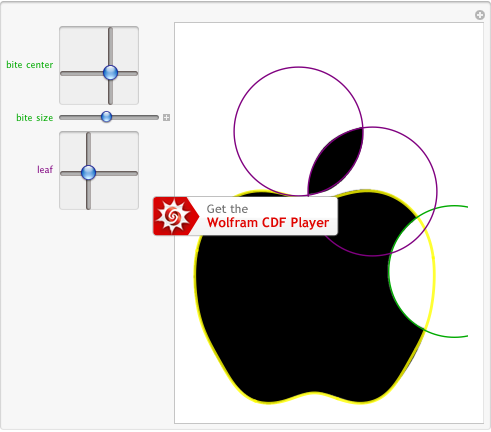So, we finally arrive at the following inequality describing the Apple logo.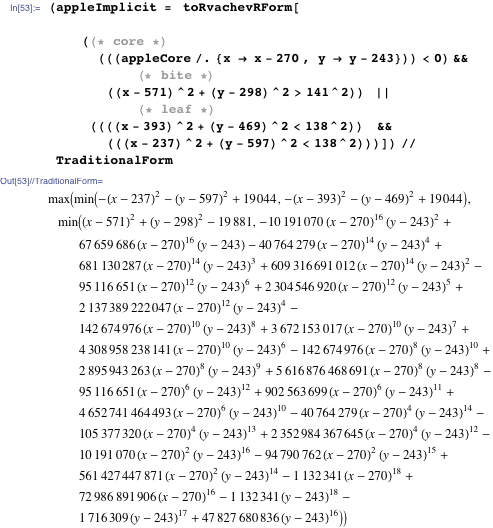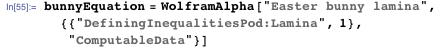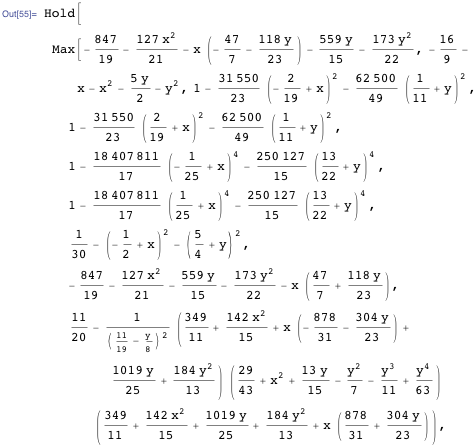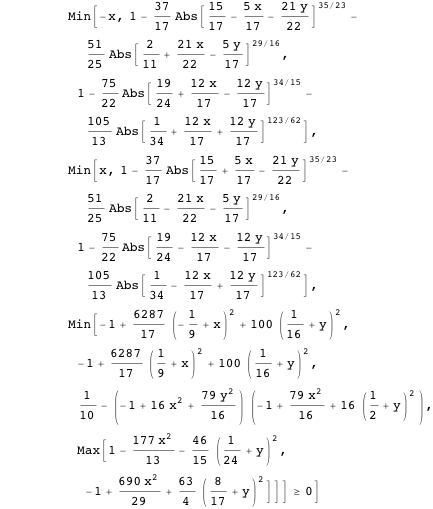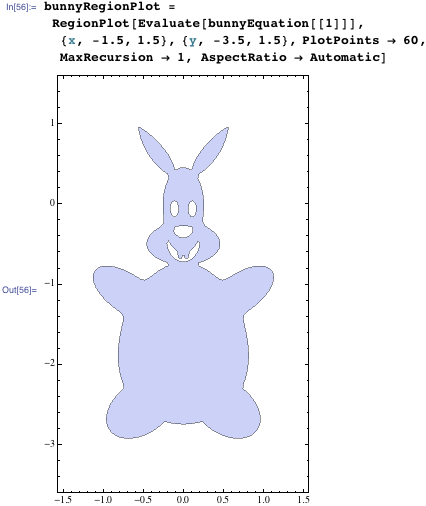We can easily extract the polygons from this 2D graphic and construct a twisted bunny in 3D.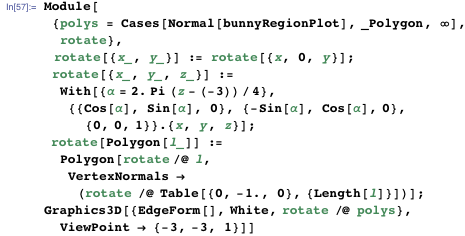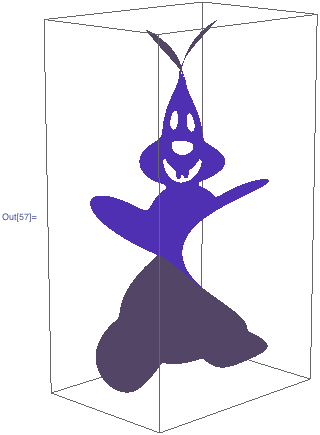The Rvachev R-form allows us to immediately make a 3D Easter bunny made from milk chocolate and strawberry-flavored chocolate. By applying the logarithm function, the parts where the defining function is negative are not shown in the 3D plot, as they give rise to complex-valued function values.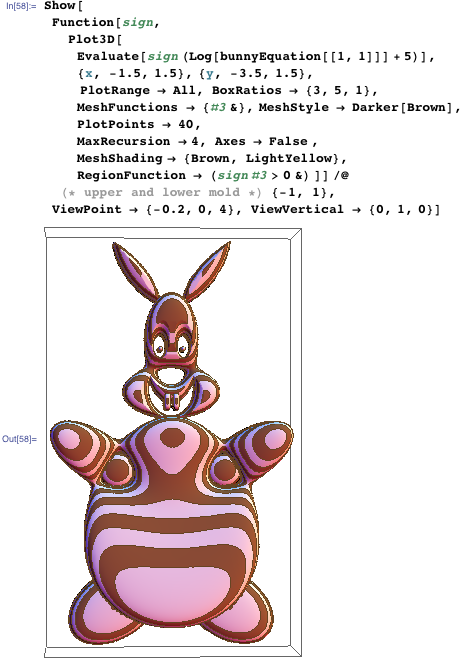We can also make the Easter bunny age within seconds, meaning his skin gets more and more wrinkles as he ages. We carry out this aging process by taking the polygons that form the lamina and letting them undergo a Brownian motion in the plane.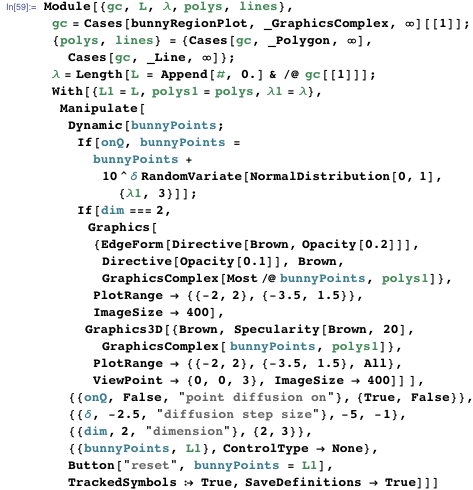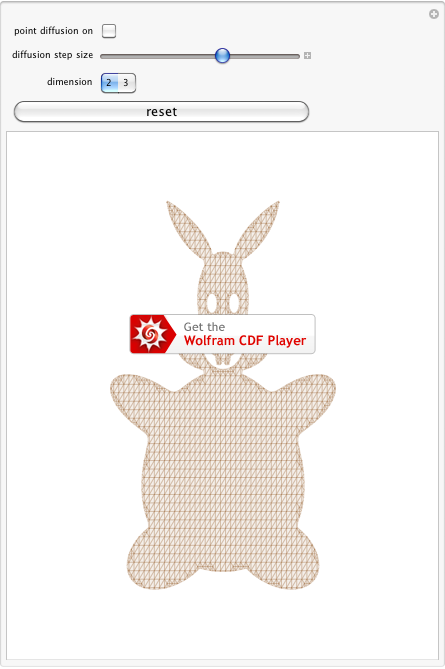Let’s now play with some car logo-like laminae. We take a Yamaha-like shape; here are the corresponding region and 3D plot.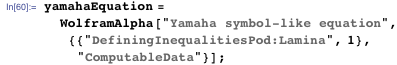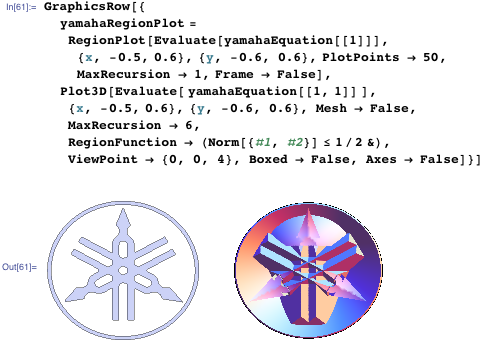We could, for instance, take the Yamaha lamina and place 3D cones in it.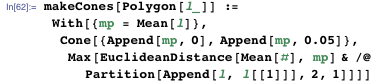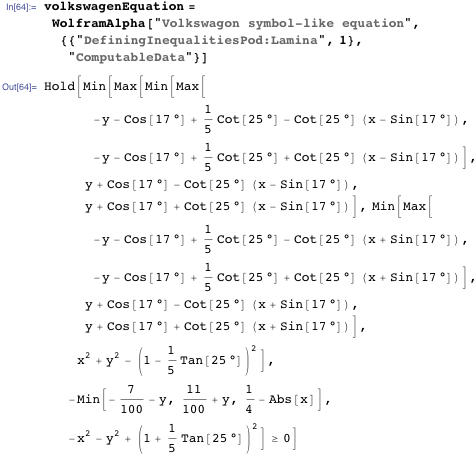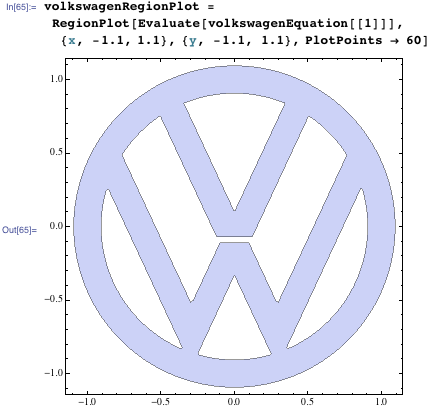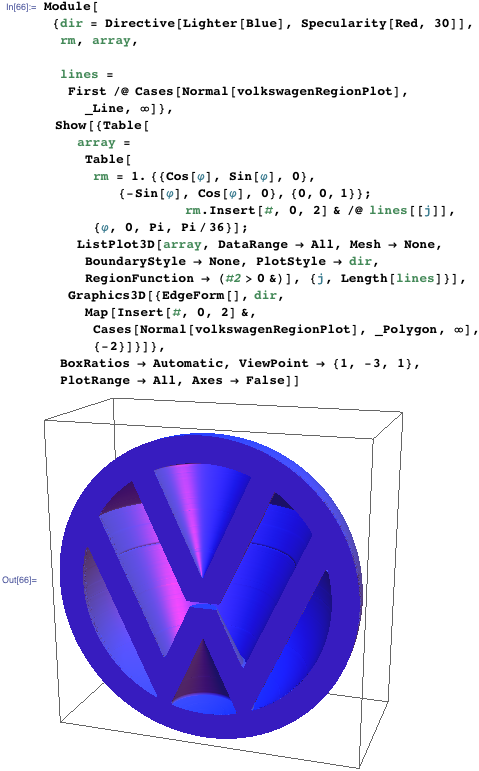By forming a weighted mixture between the Yamaha equation and the Volkswagen equation, we can form the shapes of Yamawagen and Volksmaha.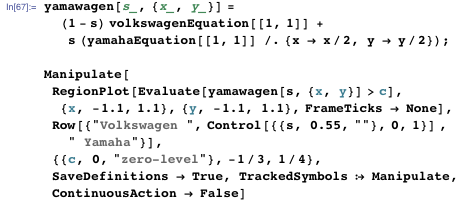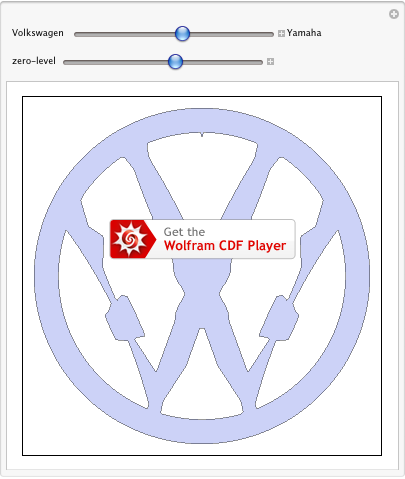Next we want to construct another, more complicated, 3D object from a 2D lamina. We take the Superman insignia.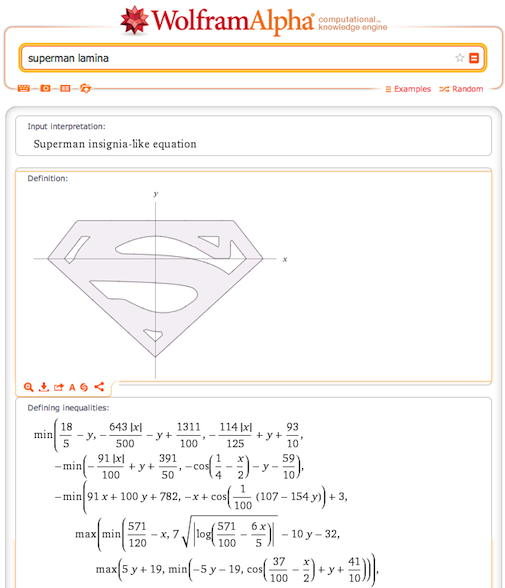The function superman[{x, y}] returns True if a point in the x, y plane is inside the insignia.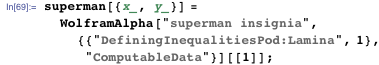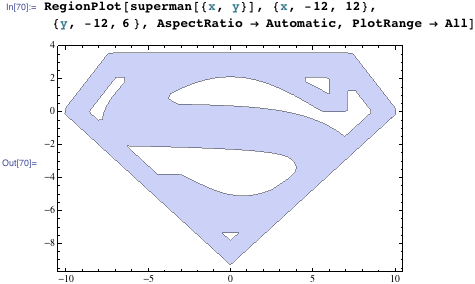And here is the object we could call the Superman solid. (Or, if made from the conjectured new supersolid state of matter, a super(man)solid.) It is straightforwardly defined through the function superman. The Superman solid engraves the shape of the Superman logo in the x, y plane as well as in the x, y plane into the resulting solid.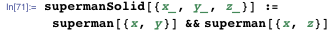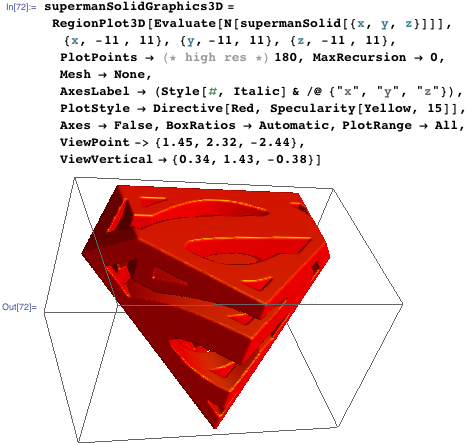Viewed from the front as well as from the side, the projection is the Superman insignia.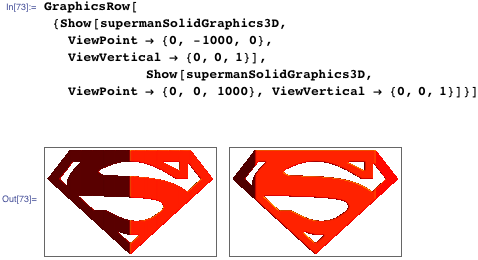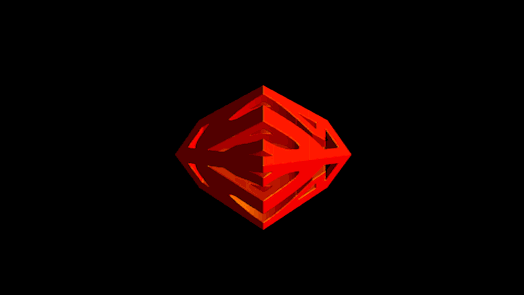To stay with the topic of Superman, we could take the Bizarro curve and roll it around to form a Bizarro-Superman cake where Superman and Bizarro face each other as cake cross sections.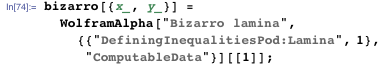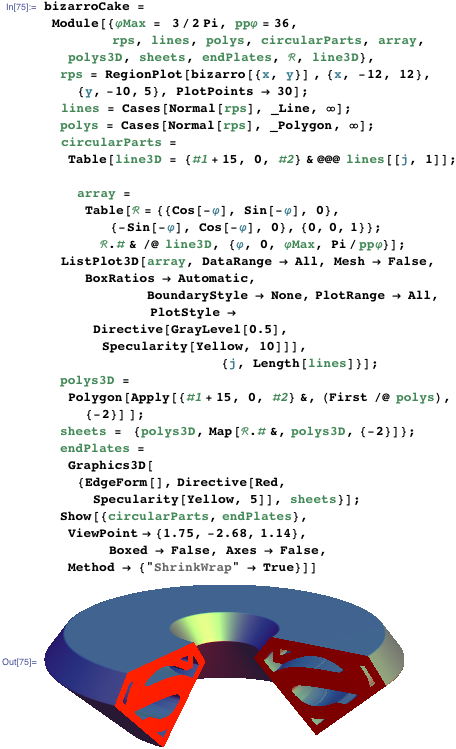This cake we can then refine by adding some kryptonite crystals, here realized through elongated triangular dipyramid polyhedra.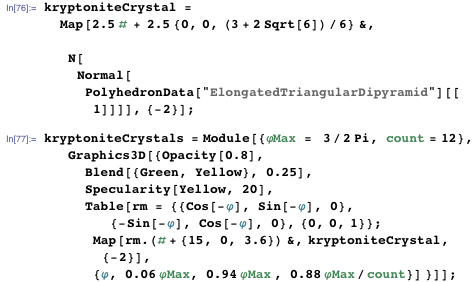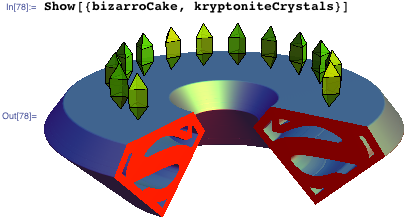Next, let’s use a Batman insignia-shaped lamina and make a quantum Batman out of it.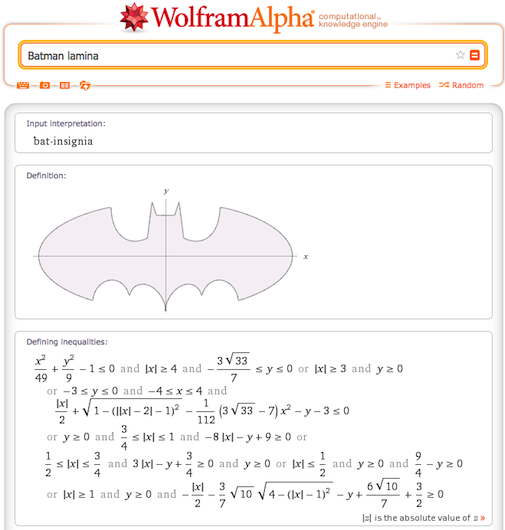We will solve the time-dependent Schrödinger equation for a quantum particle in a 2D box with the Batman insignia as the initial condition. More concretely, assume the initial wave function is 1 within the Batman insignia and 0 outside. So, the first step is the calculation of the 2D Fourier coefficients.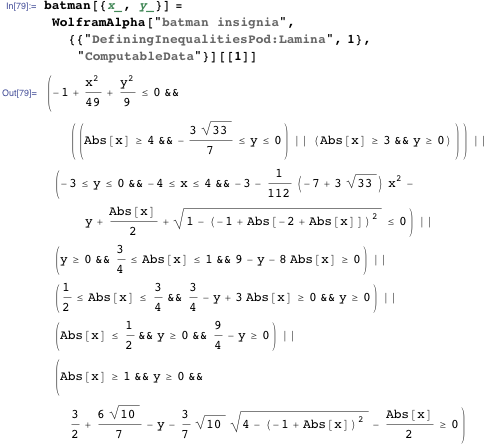Numerically integrating a highly oscillating function over a domain with sharp boundaries using numerical integration can be challenging. The shape of the Batman insignia suggests that we first integrate with respect to y and then with respect to x. The lamina can be conveniently broken up into the following subdomains.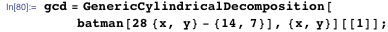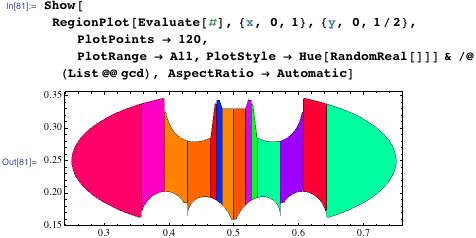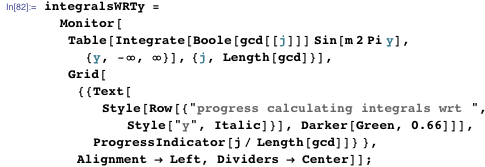All of the integrals over y can be calculated in closed form. Here is one of the integrals shown.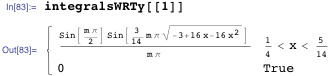To calculate the integrals over x, we need to multiply the integralsWRTy with sin(k Π x) and then integrate. Because k is the only parameter that is changing, we use the (new in Version 9) function ParametricNDSolveValue.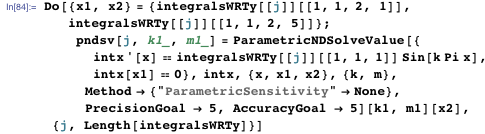We calculate 200^2 Fourier coefficients. This relatively large number is needed to obtain a good solution of the Schrödinger equation. (Due to the discontinuous nature of the initial conditions, for an accurate solution, even more modes would be needed.)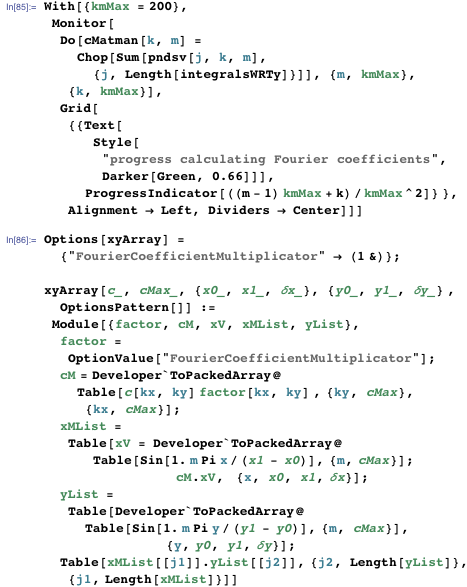Using again the function xyArray from above, here is how the Batman logo would look if it were to quantum-mechanically evolve.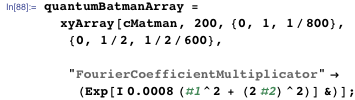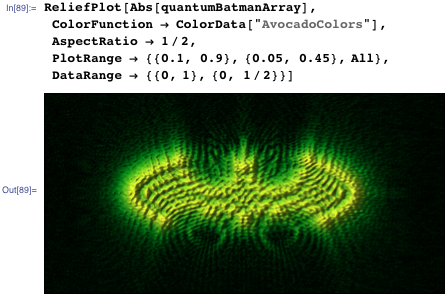We will now slowly end our brief overview on how to equationalize shapes through laminae. As a final example, we unite the Fourier series approach for curves discussed in the first blog post of this series with the Rvachev R-function approach and build an apple where the bite has the form of the silhouette of Steve Jobs, the Apple founder who suggested the name Mathematica. The last terms of the following inequality result from the Fourier series of Jobs’ facial profile.